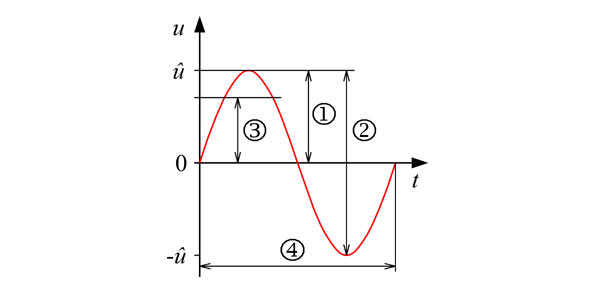# Gibilisco - Alternating-current Basics

18 Questions | Attempts: 1028
ShareSettings• 1.
Which of the following can vary with ac, but never with dc?
• A.

Power

• B.

Voltage

• C.

Frequency

• D.

Amplitude

• 2.
The length of time between a point in one cycle and the same point in the next cycle of an acwave is the
• A.

Frequency.

• B.

Magnitude.

• C.

Period.

• D.

Polarity.

• 3.
On a spectrum analyzer, an ac signal having only one frequency component looks like
• A.

A single pip.

• B.

A sine wave.

• C.

A square wave.

• D.

A sawtooth wave.

• 4.
The period of an ac wave, in seconds, is
• A.

The same as the frequency in hertz.

• B.

Not related to the frequency in any way.

• C.

Equal to 1 divided by the frequency in hertz.

• D.

Equal to the peak amplitude in volts divided by the frequency in hertz.

• 5.
The sixth harmonic of an ac wave whose period is 1.000 millisecond (1.000 ms) has afrequency of
• A.

0.006 Hz.

• B.

167.0 Hz.

• C.

7.000 kHz.

• D.

6.000 kHz.

• 6.
A degree of phase represents
• A.

6.28 cycles.

• B.

57.3 cycles.

• C.

1⁄60 of a cycle.

• D.

1⁄360 of a cycle.

• 7.
Suppose that two ac waves have the same frequency but differ in phase by exactly 1⁄20 of a cycle. What is the phase difference between these two waves?
• A.

18°

• B.

20°

• C.

36°

• D.

5.73°

• 8.
Suppose an ac signal has a frequency of 1770 Hz. What is its angular frequency?
• A.

• B.

• C.

• D.

Impossible to determine from the data given

• 9.
A triangular wave exhibits
• A.

An instantaneous rise and a defined decay.

• B.

A defined rise and an instantaneous decay.

• C.

A defined rise and a defined decay, and the two are equal.

• D.

An instantaneous rise and an instantaneous decay.

• 10.
Three-phase ac
• A.

Has sawtooth waves that add together in phase.

• B.

Consists of three sine waves in different phases.

• C.

Is a sine wave with exactly three harmonics.

• D.

Is of interest only to physicists.

• 11.
If two perfect sine waves have the same frequency and the same amplitude, but are inopposite phase, the composite wave
• A.

Has twice the amplitude of either input wave alone.

• B.

Has half the amplitude of either input wave alone.

• C.

Is complex, but has the same frequency as the originals.

• D.

Has zero amplitude (that is, it does not exist), because the two input waves cancel each other out.

• 12.
If two perfect sine waves have the same frequency and the same phase, the composite wave
• A.

Is a sine wave with an amplitude equal to the difference between the amplitudes of the two input waves.

• B.

Is a sine wave with an amplitude equal to the sum of the amplitudes of the two original waves.

• C.

Is not a sine wave, but has the same frequency as the two input waves.

• D.

Has zero amplitude (that is, it does not exist), because the two input waves cancel each other out.

• 13.
In a 117-V rms utility circuit, the positive peak voltage is approximately
• A.

+82.7 V.

• B.

+165 V.

• C.

+234 V.

• D.

+331 V

• 14.
In a 117-V rms utility circuit, the peak-to-peak voltage is approximately
• A.

82.7 V.

• B.

165 V.

• C.

234 V.

• D.

331 V.

• 15.
In a perfect sine wave, the peak-to-peak amplitude is equal to
• A.

Half the peak amplitude.

• B.

The peak amplitude.

• C.

1.414 times the peak amplitude.

• D.

Twice the peak amplitude.

• 16.
Which one of the following does not affect the power output available from a particular acgenerator?
• A.

The strength of the magnet

• B.

The number of turns in the coil

• C.

The type of natural energy source used

• D.

The speed of rotation of the coil or magnet

• 17.
If a 175-V dc source were connected in series with the utility mains from a standard walloutlet, the result would be
• A.

Smooth dc at a constant voltage.

• B.

Pure ac with equal peak voltages.

• C.

Ac with one peak voltage greater than the other.

• D.

Fluctuating dc.

• 18.
An advantage of ac over dc in utility applications is the fact that
• A.

Ac is easier to transform from one voltage to another.

• B.

Ac is transmitted with lower loss in wires.

• C.

Ac can be easily obtained from dc generators.

• D.

Ac can be generated with less-dangerous by-products.

## Related TopicsBack to top
×

Wait!
Here's an interesting quiz for you.